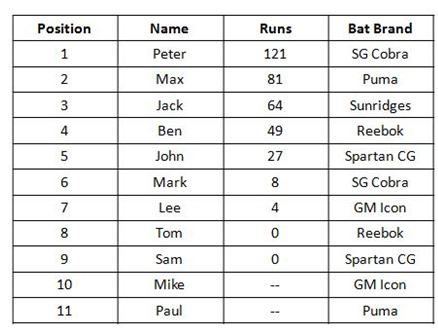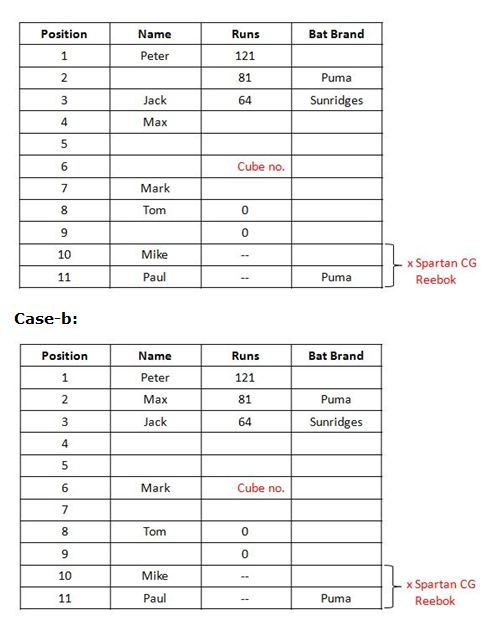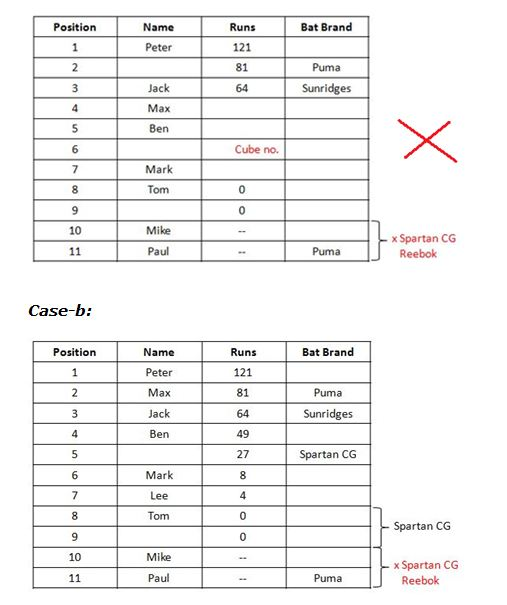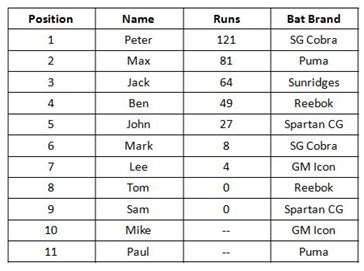# SBI PO Reasoning Questions 2019 (Day-22) High Level-New Pattern

SBI PO 2019 Notification is about to come and it is the most awaited exam among the aspirants. We all know that new pattern questions are introducing every year in the SBI PO exam. Further, the questions are getting tougher and beyond the level of the candidate’s expectations.

Our IBPS Guide is providing High-Level New Pattern Reasoning Ability Questions for SBI PO 2019 so the aspirants can practice it on a daily basis. These questions are framed by our skilled experts after understanding your needs thoroughly. Aspirants can practice these high-level questions daily to familiarize with the exact exam pattern. We wish that your rigorous preparation leads you to a successful target of becoming SBI PO.

#### “Be not afraid of growing slowly; be afraid only of standing still”

[WpProQuiz 5261]

Check here for Video Solutions (Q. 1-5)

Check here for Video Solutions (Q. 6-10)

### Click Here for SBI PO Pre 2019 High-Quality Mocks Exactly on SBI Standard

Directions (1-5): Study the following information to answer the given questions.

There are three rows given. To find out the resultant of a particular row we need to follow the following conditions: –

Condition 1: If an odd number (non-prime) is followed by another odd number (prime number) then the resultant will be the addition of both the numbers.

Condition 2: If an even number is followed by a perfect square then the resultant will be the difference between the numbers.

Condition 3: If an odd number (prime number) is followed by another odd number then the resultant will be the product of both the numbers.

Condition 4: If an even number is followed by another even number then the resultant will be the product of the numbers.

Condition 5: If an odd number (non-prime number) is followed by a perfect square number then the resultant will be the difference of both the numbers.

Condition 6: If an even number is followed by anodd number (prime) then the resultant will be the sum of both the numbers.

1) If the sum of the resultants of all three rows is 462. Then find the value of X?

13    15     X

21    64     5

36    81    23

a) 13

b) 8

c) 16

d) 9

e) None of the above

2) What will be the product of digits at unit place from resultant of all three rows?

24    31     9

23    27     49

32    17    13

a) 24

b) 16

c) 21

d) 27

e) None of the above

3) If the sum of the resultants of all three rows is 1149. Then find the square root of Y?

21    36     53

48     9      49

64    18      Y

a) 8

b) 9

c) 11

d) 10

e) None of the above

4) Find the sum of second digits from left of the resultants of all three rows?

15   9   12

20  36  17

15  23  13

a) 13

b) 8

c) 7

d) 6

e) None of the above

5) If the sum of the resultants of all three rows is 89. Then find the value of X?

24    31     X

48    9     49

20    36     17

a) 9

b) 32

c) 16

d) 12

e) None of the above

Directions (6-10): Study the following information to answer the given questions.

There was a Cricket match between Team-A and Team-B. Team -A scored 354 runs for 7 wickets. Team-A squad consists of Jack, John, Lee, Max, Mark, Ben, Mike, Paul, Sam, Peter and Tom. Each player scored different runs from 0-150 such that their score is either a square number or cube number other than zero. Not more than one player scored same runs and two players were yet to bat. Each have different brands of bat viz. Reebok, Spartan CG, Sunridges, Puma, SG Cobra and GM Icon and not less than one and more than two have same branded bat. In scorecard, the player who scored the highest runs is numbered 1 and the player who scored second highest runs is numbered 2 and so on.

Note: Sum of runs scored by all players = Total score of Team A. The players who were yet to bat also own different brands of bat and they were arranged in the scorecard in alphabetical order.

Run scored by Jack is a square of the position of Tom in the scorecard. The run scored by the 4thleast scorer (excluding the players yet to bat) is cube of a number. Reebok brand bats are used by the player who is at one of the position less than 5 and another player (who uses the same brand bat) is double the position of this player.

There are two players between Ben and the one who scored runs in a square of a number. The persons who scored runs in square and cube of a number are placed above and below of Ben respectively in scorecard. Two people owns Spartan CG bat and sum of their score is 27 runs.

Paul owns Puma bat and he is yet to bat. Neither of the players who were yet to bat owns Spartan CG nor Reebok branded bats. The runs scored by using Puma branded bat is the second highest. Lee got one of the positions in top 3 least scorers.

Lee scored runs as same as the position of Ben in scorecard and Ben scored as same as the square of position of Lee in the scorecard. Peter scored 7 runs less than the double the score of the only one who uses Sunridges brand bat.

Tom got 8thposition in scorecard without scoring any runs. Only Peter scored runs in three digits. The person who uses GM Icon brand bat is the second least among the other branded bat.

Mike is yet to bat. Number of players between Mark and Mike in the scorecard is as same the number of players between Mark and Max. Mark who is in one of the positions below John and the one who scored runs in square number as well as the one who scored runs in cube number uses SG Cobra branded bat.

Not more than one player uses Sunridges brand bat. Lee does not use SG cobra branded bat.

6) Who among the following uses Reebok brand bat?

a) Ben – Sam

b) John – Tom

c) Ben – Tom

d) Max – Lee

e) Peter – Mike

7) Who scored the 5th least runs excluding the batsman who were yet to bat?

a) Lee

b) John

c) Ben

d) Mark

e) Max

8) Which among the following Bat brand holders hold the third highest runs?

a) Sunridges

b) Reebok

c) Puma

d) Spartan CG

d) SG Cobra

9) How many players are there between Mike and John?

a) One

b) Three

c) Two

d) Four

e) More than Four

10) Which of the following statement is true?

a) Lee scored 8 runs and owns GM icon brand bat

b) Mark is three positions below Max in the scorecard

c) Tom and Ben owns same brand of bats

d) Score of Max is a cube number

e) Reebok bat holders scored second least runs.

Directions (1-5):

We have:

13    15     X

21    64     5

36    81    23

For 2nd row:

• An odd number followed by perfect square so, (64 – 21) = 43.
• Now, the row is 43 5, an odd number (prime) is followed by another odd number so, 43 x 5 = 215.

Thus, resultant of 2nd row = 215.

For 3rd row:

• An even number followed by perfect square so, (81 – 36) = 45.
• Now, the row is 45 23, an odd number (non-prime) is followed by another odd number (prime), so 45 + 23 = 68.

Thus, resultant of 3rd row = 68.

For 1st row:

• Resultant of 1st row = 462 – (215 + 68) = 179.
• An odd number (prime) followed by another odd number so, 13 x 15 = 195.
• Now, the row is 195 X, an odd number followed by X to get resultant of 179.

Thus, 195 – X = 179

X = (195 – 179) = 16, which follows Condition (3).

Hence, option C is correct choice.

We have:

24    31     9

23    27     49

32    17    13

For 1st row:

• An even number followed by an odd number (prime) so, 24 + 31 = 55.
• Now, the row is 55 9, an odd number followed by a perfect square so, (55 – 9) = 46.

Thus, resultant of 1st row = 46.

For 2nd row:

• An odd number (prime) is followed by another odd number so, (23 x 27) = 621.
• Now, the row is 621 49, an odd number followed by perfect square so, (621 – 49) = 572.

Thus, resultant of 2nd row = 572.

For 3rd row:

• An even number followed by odd number (prime) so, (32 + 17) = 49.
• Now, the row is 49 13, an odd number followed by another odd number (prime) = 62.

Thus, resultant of 3rd row = 62.

Clearly, required product = (6 x 2 x 2) = 24.

Hence, option A is correct choice.

We have:

21    36     53

48     9      49

64     Y      81

For 1st row:

• An odd number followed by perfect square so, (36 – 21) = 15.
• Now, the row is 15 53, an odd number followed by another odd number (prime) so, (53 + 15) = 68.

Thus, resultant of 1st row = 68.

For 2nd row:

• An even number followed by perfect square so, (48 – 9) = 39.
• Now, the row is 39 49, an odd number followed by perfect square number. so, (39 – 49) = 10.

Thus, resultant of 2nd row = 10.

For 3rd row:

• Resultant of 3rd row = 1149 – (10 + 86) = 1071.
• An even number followed Y thus, after applying Condition (4), we have:

(64 x 18)

• Now, the row is (64 x 18)Y, after applying Condition (1) we have:

1152 – Y = 1071

1152 – 1071 = 81

Root of 81 is 9.

Hence, option B is correct choice.

For 1st row:

• An odd number followed by perfect square so, (15 – 9) = 6.
• Now, the row is 6 12, even number is followed by another even number then the resultant will be the product of the numbers so, (6 x 12) = 72.

Thus, resultant of 1st row = 72.

For 2nd row:

• An even number followed by perfect square so, (36 – 20) = 16.
• Now, the row is 16 17, an even number followed by odd number. So, (16+17) = 33.

Thus, resultant of 2nd row = 33.

For 3rd row:

• An odd number followed by another odd number so, (15 +23) = 38.
• Now, the row is 3813, even number is followed by an odd (prime) number so, (38+13) = 51.

Thus, resultant of 3rd row = 51.

Clearly, 2+3+1=6.

Hence option D is the correct answer.

For 1st row:

• An even number followed by an odd number (prime) so, 24 + 31 = 55.
• Now, the row is 55 X

For 2nd row:

• An even number followed by perfect square so, (48 – 9) = 39.
• Now, the row is 39 49, an odd number followed by perfect square number. So, (39 – 49) = 10.

Thus, resultant of 2nd row = 10.

For 2nd row:

• An even number followed by perfect square so, (36 – 20) = 16.
• Now, the row is 16 17, an even number followed by odd number. So, (16+17) = 33.

Thus, resultant of 2nd row = 33.

So, 89-10-33 = 46 (resultant of row1)

55 – X = 46

So, the value of X is 9.

Hence, option A is correct choice.

Directions (6-10):• Run scored by Jack is a square of the position of Tom in the scorecard. The run scored by the 4th least scorer (excluding the players yet to bat) is a cube number.

So we can fix that the runs scored by the player in 6th position is a cube number.

• Paul owns Puma bat and he is yet to bat. Neither of the players who were yet to bat owns Spartan CG nor Reebok branded bats.
• The runs scored by using Puma branded bat is the second highest.

So we can conclude that the player in 2nd position owns Puma brand bat.

• Tom got 8th position in scorecard without scoring any runs. Only Peter scored runs in three digits.
• Peter scored 7 runs less than the double the score of the only one who uses Sunridges brand bat.

As Peter scored in three digits he must be the highest scorer and the only possible score of Sunridges is 64 which can be concluded as Jack’s score.

• Mike, who is yet to bat, is in one of the positions below Mark in the scorecard which is as same as the position of Max above Mark in the scorecard.

Already we found that Paul was yet to bat and now Mike gets added. So, we can conclude the position of both based on the alphabetical order i.e. Paul in 11th Position and Mike in 10th position.

We get two possibilities Case-a and Case-b based on the position of Mark.

Case-a:• There are two players between Ben and the one who scored runs in a square number; both from above and below of Ben.
• Lee scored runs as same as the position of Ben in scorecard and Ben scored as same as the square of position of Lee in the scorecard.
• The players who are in one of the position above 5 and double the same position uses Reebok brand bat.
• Two people owns Spartan CG bat and sum of their score is 27 runs.
• Lee got one of the positions in top 3 least scorers.

We can fix the position of Ben as 4 not as 5 or 6, because Position of Lee cannot be 5 and 6 which are neither a cube nor square number. So Case-a gets eliminated.

As the position of Ben is fixed, we can conclude the position of Lee as 7. And the score of Ben is 49 runs and Lee is 4 runs. Now the remaining runs in 354 is 35 runs in which one must be a cube number. We get only one possibility 27 and 8, so we can enter the run of 5th position as 27 and Mark as 8 runs.

As Spartan CG bat users scored 27 runs it must be 27 + 0, so we can conclude Spartan CG bat user in 5th position and either in 8th or 9th position.

Case-a:• Sums of runs scored by using GM Icon brand bat is the least among other branded bat.
• Mark who is below John and the one who scored runs in square number uses SG Cobra branded bat.

We can conclude that Ben and Tom has Reebok brand bat.  We can conclude the position of John and Peter’s bat brand from the above statements.

Case b: